# Mechanical Engineering - Hydraulics and Fluid Mechanics

### Exercise :: Hydraulics and Fluid Mechanics - Section 2

16.

The specific gravity has no units.

 A. Agree B. Disagree

Answer: Option A

Explanation:

No answer description available for this question. Let us discuss.

17.

The depth of water in a channel corresponding to the minimum specific energy is known as critical depth.

 A. Agree B. Disagree

Answer: Option A

Explanation:

No answer description available for this question. Let us discuss.

18.

The ratio of the inertia force to the gravity force is called Froude number.

 A. Agree B. Disagree

Answer: Option A

Explanation:

No answer description available for this question. Let us discuss.

19.

Which of the following statement is wrong?

 A. A flow whose streamline is represented by a curve, is called two dimensional flow. B. The total energy of a liquid particle is the sum of potential energy, kinetic energy and pressure energy. C. The length of divergent portion in a venturimeter is equal to the convergent portion. D. A pitot tube is used to measure the velocity of flow at the required point in a pipe.

Answer: Option C

Explanation:

No answer description available for this question. Let us discuss.

20.

A vertically immersed surface is shown in the below figure. The distance of its centre of pressure from the water surface is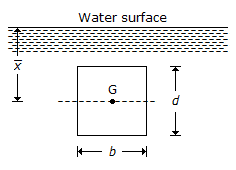A.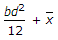B.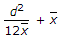C.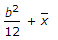D.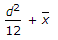Answer: Option B

Explanation:

No answer description available for this question. Let us discuss.

#### Current Affairs 2021

Interview Questions and Answers